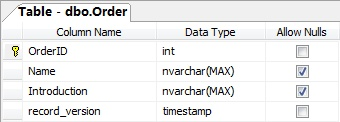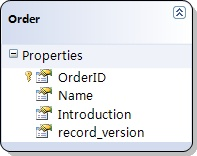# 在Linq to Sql中管理并发更新时的冲突（3）：使用记录的时间戳进行检测

 使用时间戳1
 使用时间戳2
 使用时间戳3```try
{
LinqToSqlDemoDataContext dataContext = new LinqToSqlDemoDataContext();

Order order = dataContext.Orders.Single(o => o.OrderID == 1);
order.Name = "New Order Name";

dataContext.Log = Console.Out;
// 在下面的语句上设置一个断点
dataContext.SubmitChanges();
}
catch (ChangeConflictException e)
{
Console.WriteLine(e.Message);
}

```UPDATE [dbo].[Order]
SET [Name] = @p2
WHERE ([OrderID] = @p0) AND ([record_version] = @p1)

SELECT [t1].[record_version]
FROM [dbo].[Order] AS [t1]
WHERE ((@@ROWCOUNT) > 0) AND ([t1].[OrderID] = @p3)
-- @p0: Input Int (Size = 0; Prec = 0; Scale = 0) 
-- @p1: Input Timestamp (Size = 8; Prec = 0; Scale = 0) [SqlBinary(8)]
-- @p2: Input NVarChar (Size = 14; Prec = 0; Scale = 0) [New Order Name]
-- @p3: Input Int (Size = 0; Prec = 0; Scale = 0) 
-- Context: SqlProvider(Sql2005) Model: AttributedMetaModel Build: 3.5.21022.8```

0
0